RS Aggarwal Class 7 Solutions Chapter 6 - Algebraic Expressions

RS Aggarwal Class 7 Chapter 6 - Algebraic Expressions Solutions Free PDF

An expression made up with integer variables and constants with algebraic operations such as multiplication, addition, subtraction and division is called as an algebraic expression. These expressions are also useful for solving the roots of a polynomial and is useful in obtaining the solutions of a polynomial equation

The unique terminology employed by algebraic expressions are:

1. Exponents
2. Coefficient
3. Term
4. Operator
5. Constant
6. Variables

Get the RS Aggarwal Class 7 Solutions Algebraic Expressions below: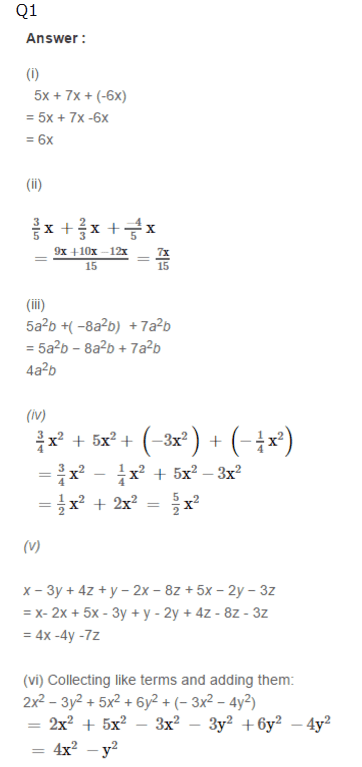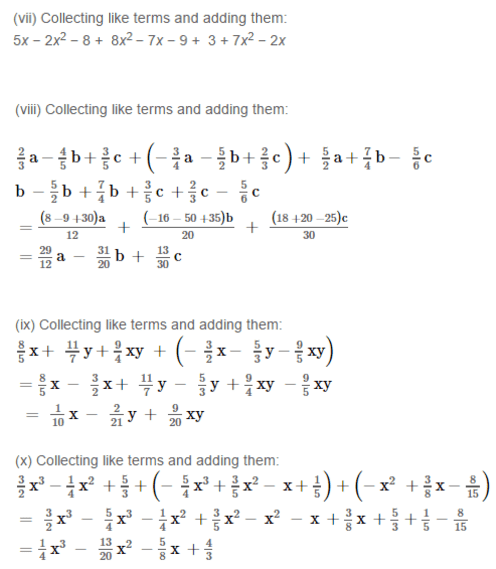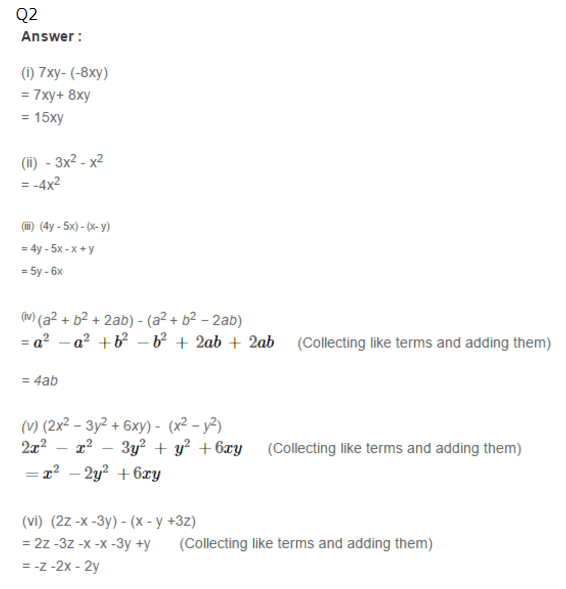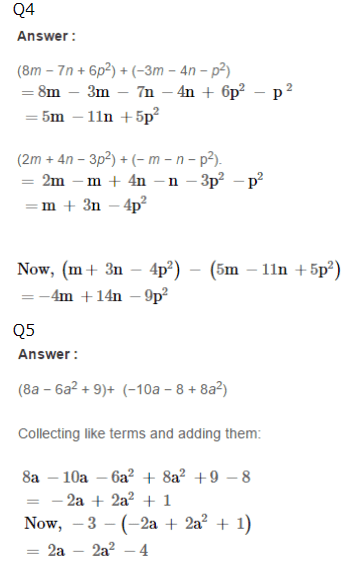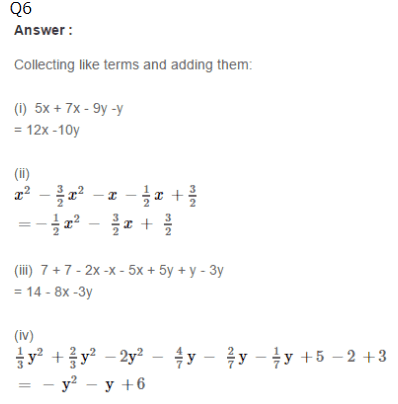Practise This Question

Electric bell works on which of the following effects of current?